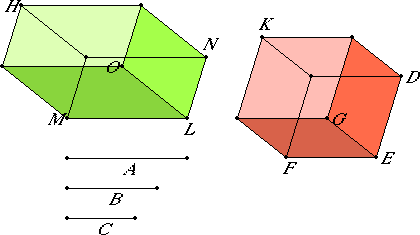# Proposition 36

If three straight lines are proportional, then the parallelepipedal solid formed out of the three equals the parallelepipedal solid on the mean which is equilateral, but equiangular with the aforesaid solid.

Let A, B, and C be three straight lines in proportion, so that A is to B as B is to C.

I say that the solid formed out of A, B, and C equals the solid on B which is equilateral, but equiangular with the aforesaid solid.I.3

Set out the solid angle at E contained by the angles DEG, GEF, and FED, and make each of the straight lines DE, GE, and EF equal to B. Complete the parallelepipedal solid EK. Make LM equal to A. Construct a solid angle at the point L on the straight line LM equal to the solid angle at E, namely that contained by NLO, OLM, and MLN. Make LO equal to B, and LN equal to C.

VI.4

Now, since A is to B as B is to C, while A equals LM, and B equals each of the straight lines LO, ED, and C to LN, therefore LM is to EF as DE is to LN. Thus the sides about the equal angles NLM, DEF are reciprocally proportional, therefore the parallelogram MN equals the parallelogram DF.

XI.35,Cor

And, since the angles DEF and NLM are two plane rectilinear angles, and on them the elevated straight lines LO and EG are set up which equal one an other and contain equal angles with the original straight lines respectively, therefore the perpendiculars drawn from the points G and O to the planes through NL and LM and through DE and EF equal one another, therefore the solids LH and EK are of the same height.

XI.31

But parallelepipedal solids on equal bases and of the same height equal one another, therefore the solid HL equals the solid EK.

And LH is the solid formed out of A, B, and C, and EK is the solid on B, therefore the parallelepipedal solid formed out of A, B, and C equals the solid on B which is equilateral, but equiangular with the aforesaid solid.

Therefore, if three straight lines are proportional, then the parallelepipedal solid formed out of the three equals the parallelepipedal solid on the mean which is equilateral, but equiangular with the aforesaid solid.

Q.E.D.

## Guide

This straightforward proof depends on viewing the two parallelepipeds as having the same height, which they do if the base of the first is taken to be the parallelogram MN and the base of the second the parallelogram DF.